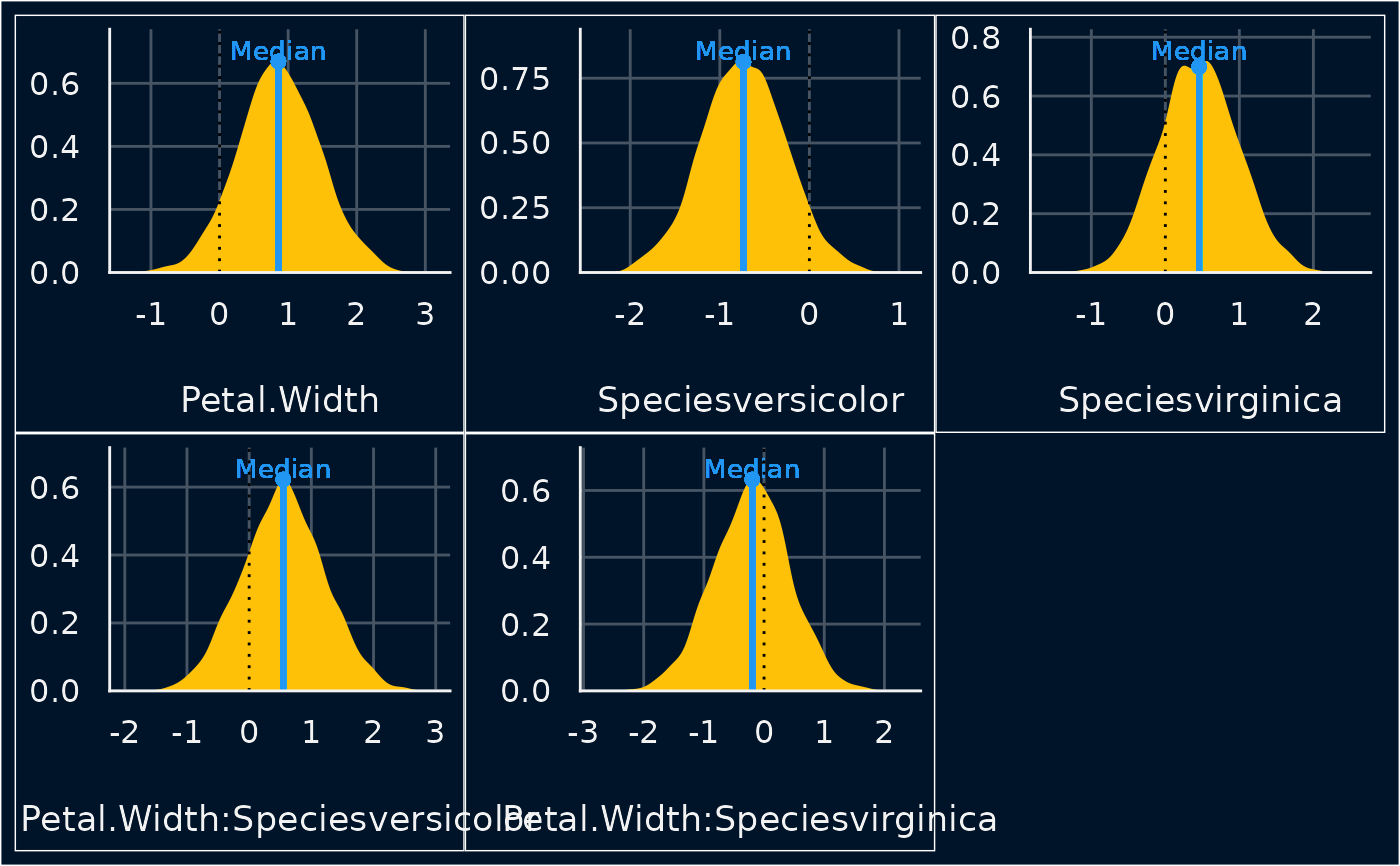The plot() method for the bayestestR::point_estimate().

## Usage

# S3 method for see_point_estimate
plot(
x,
data = NULL,
size_point = 2,
size_text = 3.5,
panel = TRUE,
show_labels = TRUE,
show_intercept = FALSE,
priors = FALSE,
priors_alpha = 0.4,
...
)

## Arguments

x

An object.

data

The original data used to create this object. Can be a statistical model.

size_point

Numeric specifying size of point-geoms.

size_text

Numeric value specifying size of text labels.

panel

Logical, if TRUE, plots are arranged as panels; else, single plots are returned.

show_labels

Logical. If TRUE, the text labels for the point estimates (i.e. "Mean", "Median" and/or "MAP") are shown. You may set show_labels = FALSE in case of overlapping labels, and add your own legend or footnote to the plot.

show_intercept

Logical, if TRUE, the intercept-parameter is included in the plot. By default, it is hidden because in many cases the intercept-parameter has a posterior distribution on a very different location, so density curves of posterior distributions for other parameters are hardly visible.

priors

Logical. If TRUE, prior distributions are simulated (using bayestestR::simulate_prior()) and added to the plot.

priors_alpha

Numeric value specifying alpha for the prior distributions.

...

Arguments passed to or from other methods.

## Value

A ggplot2-object.

## Examples

# \donttest{
if (require("bayestestR") && require("rstanarm")) {
set.seed(123)
m <- stan_glm(Sepal.Length ~ Petal.Width * Species, data = iris, refresh = 0)
result <- point_estimate(m, centrality = "median")
result
plot(result)
}# }